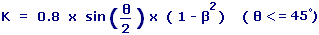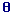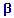Pipe Contraction Calculator Enter value and click on calculate. Result will be displayed.= Angle of Approach= dminor / dmajor
 Enter your values: Angle of Approach: (Enter Angle Less than 180°): Result: K value:

 Web www.calculatoredge.com
 A change in fitting diameter causes a pressure loss due to the change in velocity of the fluid as it passes through the fitting. The pressure drop is also dependent on the rate at which the change of direction in the fitting occurs.

This formula is used to calculate the K value for pipe contractions.LINKSDISCLAIMERCONTACT US